# Assignments

Quadratic functions are frequently used in mathematics. Therefore it is important to know:

• that the graph of a quadratic function is a parabola;
• when the parabola opens up or down;
• how you can quickly determine the intersection point with the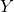-axis; and
• how you can find out whether the parabola has no, one or two intersection points with the-axis and that the discriminant plays an important role.

1. Given the function:1. What is the intersection point of the graph and the-axis?
2. Do the graph and the-axis have intersection points, and if so, how many?
3. What is the symmetry axis?

Solution

2. Given the function: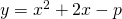1. For which value of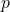does the parabola have no intersection points with the-axis?
2. Calculate the intersection point with the-axis.
3. Does the graph has a maximum or a minimum?

Solution

3. Given the function: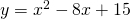1. What is the intersection point of the graph with the-axis?
2. Does the graph have intersection points with the-axis, and if so, how many? Calculate the coordinates.
3. What is the symmetry axis?

Solution

4. The equation of a parabola is: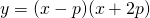For which value ofdoes the top of this parabola lie on the line:Solution

5. The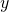-coordinate of the top of the parabola: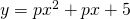is equal to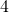.

Calculate.

Solution

6. For which value ofdoes the parabola: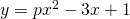have no intersection points with the-axis?

Solution

7. For which value ofdoes the parabola: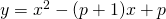have two intersection points with the-axis having a mutual distance of?

Solution

8. For which value ofdoes the top of the parabola:lie on the line: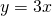Solution

9. Calculate the equation of the parabola going through the points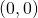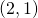and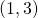.

Solution

10. For which value ofdo the parabolas: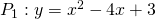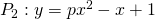have just one point in common.

Solution

0